Published

Purdue Verti-Fix

Our device is for primary care physicians as well as at home patients to treat Benign Paroxysmal Positional Vertigo with ease.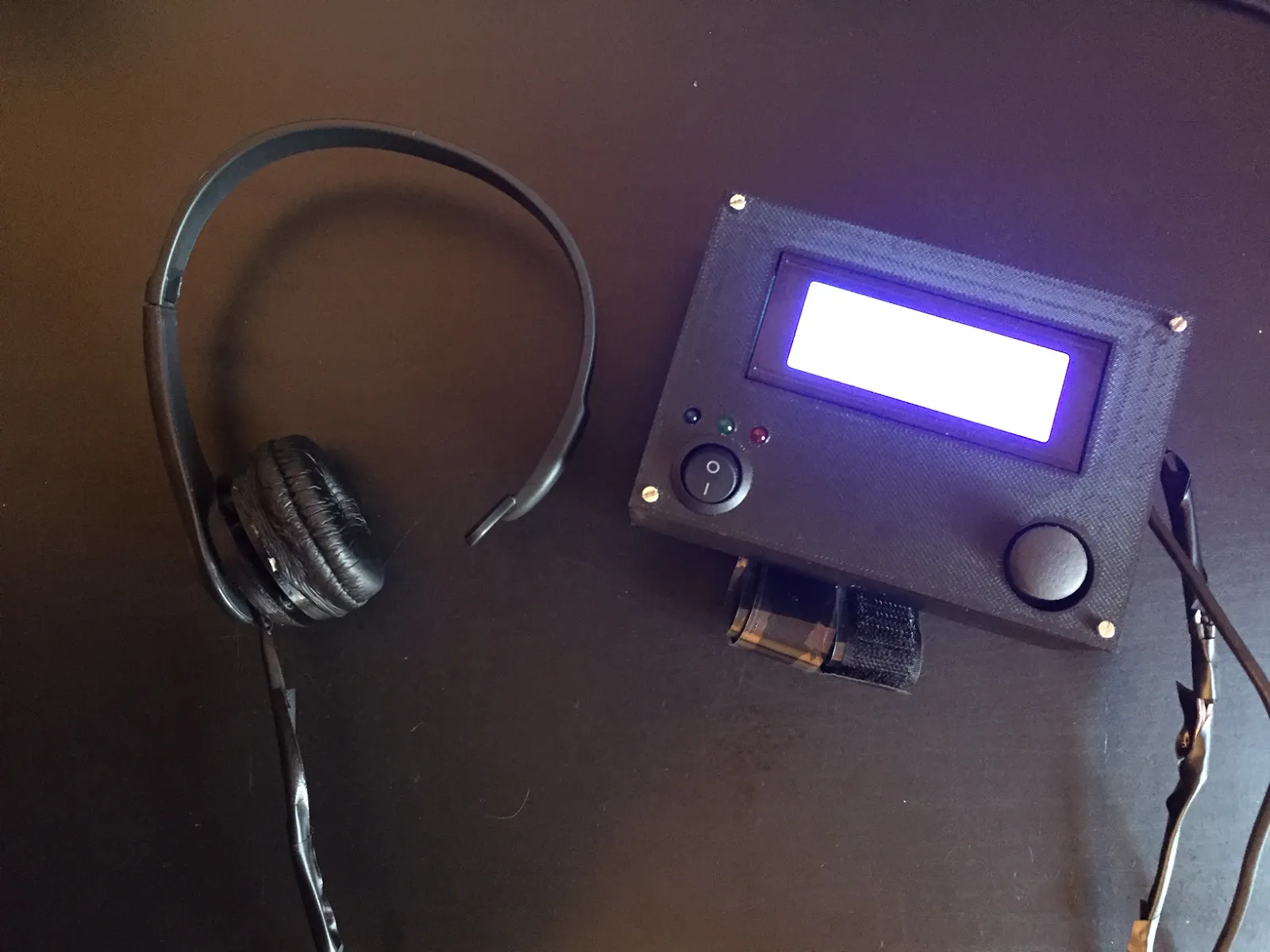Things used in this project

Hardware components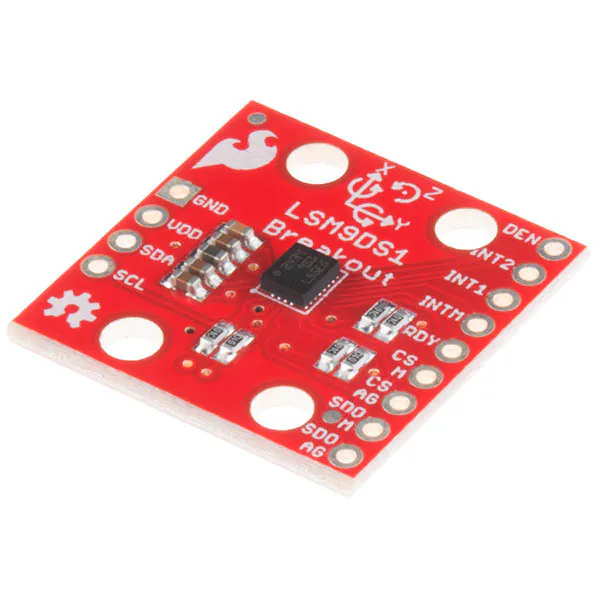SparkFun 9 Degrees of Freedom IMU Breakout - LSM9DS1
×1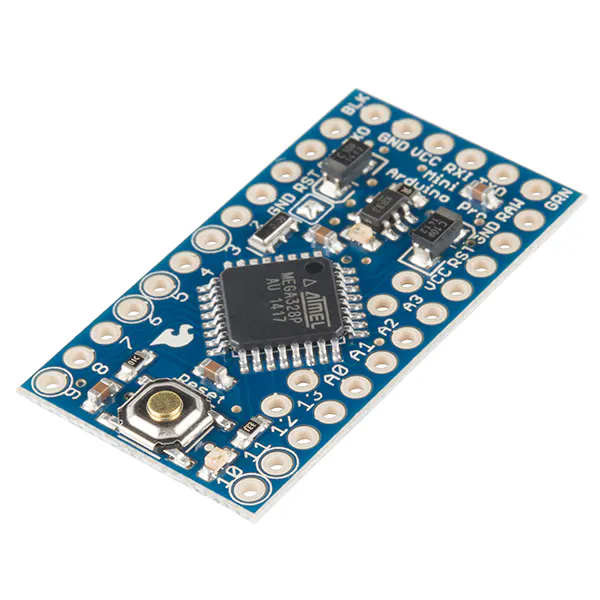SparkFun Arduino Pro Mini 328 - 3.3V/8MHz
×1LED (generic)
×3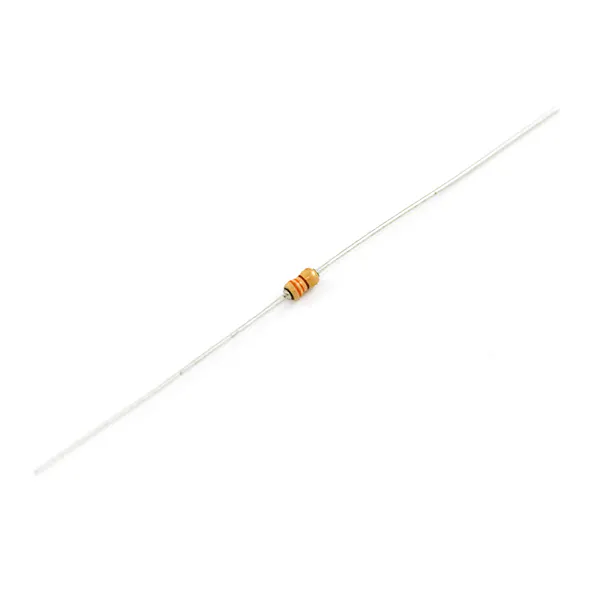Resistor 330 ohm
×3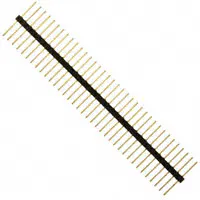Male-Header 36 Position 1 Row- Long (0.1")
×1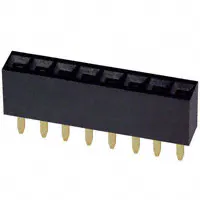Female Header 8 Position 1 Row (0.1")
×6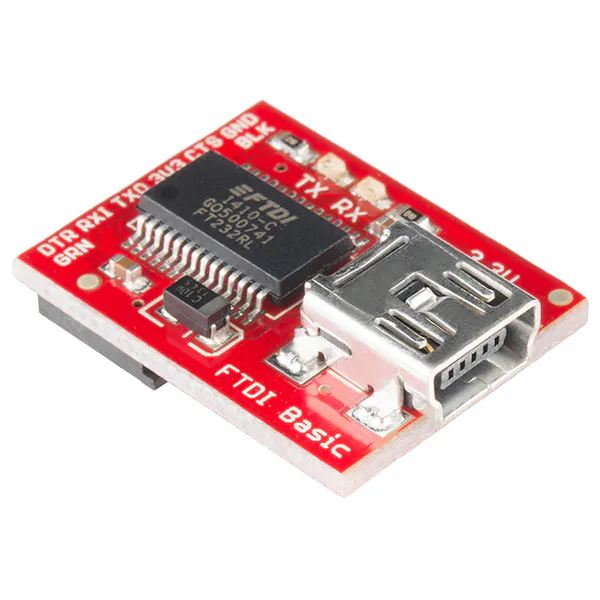SparkFun FTDI Basic Breakout - 3.3V
×1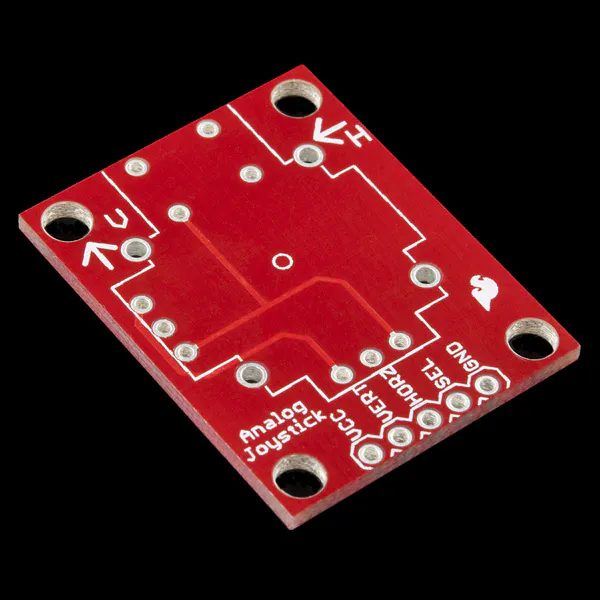SparkFun Thumb Joystick Breakout
×1
 SparkFun Thumb Joystick
×1
 Sony PSEye Camera
×1
 Sony PSEye Camera
×1
 M12 Lens
×1
 M12 Lens Mount
×1
 IR LEDs
×36
 MOSFET N-CH 60V 60A TO-220
×2
 MOSFET N-CH 800V 2.5A
×2
 MOSFET N-CH 75V 120A TO-220
×2Resistor 10k ohm
×1
 Voltage Regulator
×1
 Rocker Switch
×1
 20x4 LCD
×1

Software apps and online servicesArduino IDE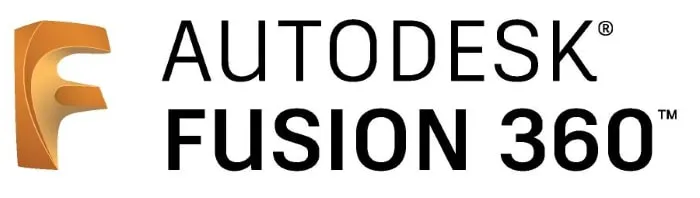Autodesk Fusion 360Hand tools and fabrication machinesSoldering iron (generic)

Custom parts and enclosures

Top Lid

Central Console Top Enclosure

Schematics

Eyewriter Schematic

From the Instructables Eyewriter 2.0, we obtained and used this schematic along with the one from Hikari Blogspot to build our eye tracker.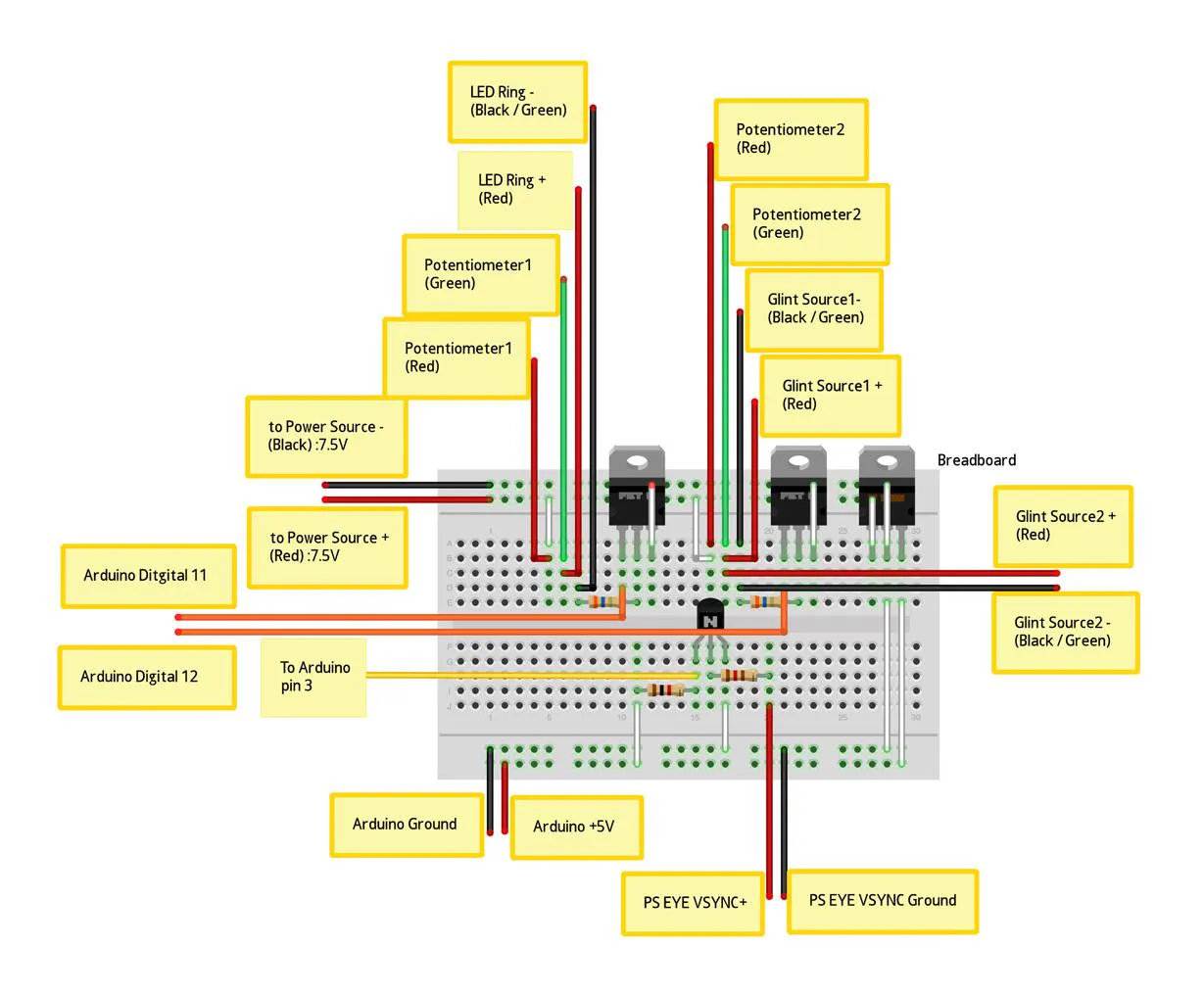Maneuvers Schematic

This is the schematic for the device that ensures movements are carried out properly.

Eyetracking Schematic

Includes the PSEye, the ring of infrared LEDs, and the Arduino. This was taken from http://hikarielectronics.blogspot.com/ which details how to build the Eyewriter 2.0 with the PSEye camera.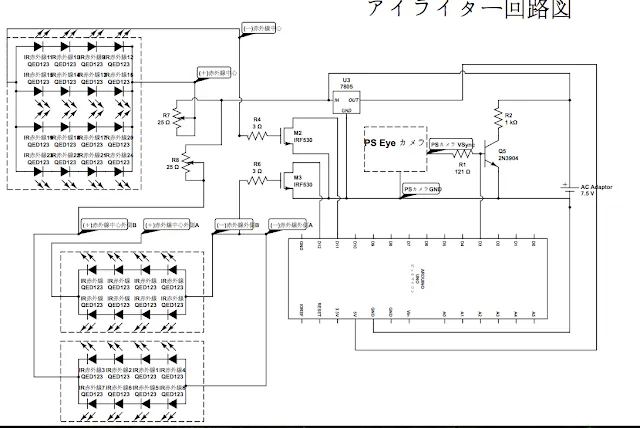Code

Arduino Programming

Arduino
This code runs on the microcontroller to carry out the maneuvers required to treat BPPV Vertigo.
#include <Wire.h>
#include <SPI.h>
#include <SparkFunLSM9DS1.h>
#include <Integrator.h>
#include <Wire.h>
#include <LiquidCrystal_I2C.h>

LSM9DS1 imu;
LiquidCrystal_I2C lcd(0x27, 20, 4);

#define LSM9DS1_M	0x1E // Would be 0x1C if SDO_M is LOW
#define LSM9DS1_AG	0x6B // Would be 0x6A if SDO_AG is LOW

// x - 0
// y - 1
// z - 2
#define FFA 1
#define RFA 2
#define UFA 0

#define GET_FFA(IMU) (FFA == 0 ? IMU.ax : (FFA == 1 ? IMU.ay : (FFA == 2 ? IMU.az : 0)))
#define GET_RFA(IMU) (RFA == 0 ? IMU.ax : (RFA == 1 ? IMU.ay : (RFA == 2 ? IMU.az : 0)))
#define GET_UFA(IMU) (UFA == 0 ? IMU.ax : (UFA == 1 ? IMU.ay : (UFA == 2 ? IMU.az : 0)))

#define GET_FFG(IMU) (FFA == 0 ? IMU.gx : (FFA == 1 ? IMU.gy : (FFA == 2 ? IMU.gz : 0)))
#define GET_RFG(IMU) (RFA == 0 ? IMU.gx : (RFA == 1 ? IMU.gy : (RFA == 2 ? IMU.gz : 0)))
#define GET_UFG(IMU) (UFA == 0 ? IMU.gx : (UFA == 1 ? IMU.gy : (UFA == 2 ? IMU.gz : 0)))

#define GET_FFM(IMU) (FFA == 0 ? IMU.mx : (FFA == 1 ? IMU.my : (FFA == 2 ? IMU.mz : 0)))
#define GET_RFM(IMU) (RFA == 0 ? IMU.mx : (RFA == 1 ? IMU.my : (RFA == 2 ? IMU.mz : 0)))
#define GET_UFM(IMU) (UFA == 0 ? IMU.mx : (UFA == 1 ? IMU.my : (UFA == 2 ? IMU.mz : 0)))

#define POSITION_MET 9
#define LED2 8
#define LED3 7

#define PROGRAM_BUTTON 6
#define SAVE_STATE_BUTTON 5

struct Measurement{
double front_facing;
double right_facing;
double up_facing;
};

enum PositionMatch{
POSITION_MATCH,
POSITION_NOT_MATCH,
POSITION_UNSURE,
};

enum PositionType{
POSITION_SITTING,
POSITION_LAYING,
};

Measurement gyro_reference{0, 0, 0};

#define MEASUREMENT_CYCLES 1
Measurement takeMeasurementAccel(int measurement_cycles)
{
Measurement average {0, 0, 0};

for(int i = 0; i < measurement_cycles; i++){
if ( imu.accelAvailable() )
{
}
/*
vector g_raw(imu.calcAccel(imu.ax), imu.calcAccel(imu.ay), imu.calcAccel(imu.az));
*/
average.front_facing += imu.calcAccel(GET_FFA(imu));
average.right_facing += imu.calcAccel(GET_RFA(imu));
average.up_facing += imu.calcAccel(GET_UFA(imu));
}

average.front_facing /= measurement_cycles;
average.right_facing /= measurement_cycles;
average.up_facing /= measurement_cycles;

return average;
}

Measurement takeMeasurementAccel(){
return takeMeasurementAccel(MEASUREMENT_CYCLES);
}

Measurement takeMeasurementGyro(int measurement_cycles)
{
Measurement average {0, 0, 0};

for(int i = 0; i < measurement_cycles; i++){
if ( imu.gyroAvailable() )
{
}
/*
vector g_raw(imu.calcAccel(imu.ax), imu.calcAccel(imu.ay), imu.calcAccel(imu.az));
*/
average.front_facing += imu.calcGyro(GET_FFG(imu));
average.right_facing += imu.calcGyro(GET_RFG(imu));
average.up_facing += imu.calcGyro(GET_UFG(imu));
}

average.front_facing /= measurement_cycles;
average.right_facing /= measurement_cycles;
average.up_facing /= measurement_cycles;

average.front_facing -= gyro_reference.front_facing;
average.right_facing -= gyro_reference.right_facing;
average.up_facing -= gyro_reference.up_facing;

return average;
}

Measurement takeMeasurementGyro(){
return takeMeasurementGyro(MEASUREMENT_CYCLES);
}

Measurement takeMeasurementMag(int measurement_cycles)
{
Measurement average {0, 0, 0};

for(int i = 0; i < measurement_cycles; i++){
if ( imu.magAvailable() )
{
}
/*
vector g_raw(imu.calcAccel(imu.ax), imu.calcAccel(imu.ay), imu.calcAccel(imu.az));
*/
average.front_facing += imu.calcMag(GET_FFM(imu));
average.right_facing += imu.calcMag(GET_RFM(imu));
average.up_facing += imu.calcMag(GET_UFM(imu));
}

average.front_facing /= measurement_cycles;
average.right_facing /= measurement_cycles;
average.up_facing /= measurement_cycles;

return average;
}

Measurement takeMeasurementMag(){
return takeMeasurementMag(MEASUREMENT_CYCLES);
}

void calibrateGyro(){
gyro_reference = takeMeasurementGyro(100);
}

#define JOYSTICK_BUTTON 6
#define JOYSTICK_HORIZONTAL A0
#define JOYSTICK_VERTICAL A1

//MUST BE ZERO INITIALIZED!!!

void setup()
{
lcd.init();
lcd.backlight();

pinMode(JOYSTICK_BUTTON, INPUT);
pinMode(JOYSTICK_HORIZONTAL, INPUT);
pinMode(JOYSTICK_VERTICAL, INPUT);

Serial.begin(115200);
Serial.setTimeout(0);

// Before initializing the IMU, there are a few settings
// we may need to adjust. Use the settings struct to set
// the device's communication mode and addresses:
imu.settings.device.commInterface = IMU_MODE_I2C;
// The above lines will only take effect AFTER calling
// imu.begin(), which verifies communication with the IMU
// and turns it on.
if (!imu.begin())
{
Serial.println("Failed to communicate with LSM9DS1.");
Serial.println("Double-check wiring.");
Serial.println("Default settings in this sketch will " \
"work for an out of the box LSM9DS1 " \
"Breakout, but may need to be modified " \
"if the board jumpers are.");
while (1)
;
}
//Wire.setClock(400000);
/*
reference_chin = getDegreesChinTilt();
reference_neck = getDegreesNeck();
reference_spin = getMaxSpin();
*/
/*
getChinTilt = &getDegreesChinTilt;
getNeckTilt = &getDegreesNeck;
*/
/*
getGyroData = &takeMeasurementGyro;
getAccelData = &takeMeasurementAccel;
*/
calibrateGyro();
}
/*
double getDegreesNeck(const Measurement& m){
return atan2(m.right_facing, pow(pow(m.front_facing, 2) + pow(m.up_facing, 2), .5)) * 180.0 / M_PI;
}

double calcDegreesChinTilt(const Measurement& m){
return atan2(m.up_facing, pow(pow(m.front_facing, 2) + pow(m.right_facing, 2), .5)) * 180.0 / M_PI;
}
double getDegreesNeck(){
return getDegreesNeck(takeMeasurementAccel()) - reference_neck;
}

double getDegreesChinTilt(){
return calcDegreesChinTilt(takeMeasurementAccel()) - reference_chin;
}
*/
double calcMaxSpin(const Measurement& m){
if(m.front_facing > m.right_facing && m.front_facing > m.up_facing)
return m.front_facing;
else if(m.right_facing > m.front_facing && m.right_facing > m.up_facing)
return m.right_facing;
else if(m.up_facing > m.front_facing && m.up_facing > m.right_facing)
return m.up_facing;
else
return m.up_facing;
}

double getMaxSpin(){
return calcMaxSpin(takeMeasurementGyro()) - calcMaxSpin(gyro_reference);
}

double compass(){
Measurement m = takeMeasurementMag();
/*
Serial.print(m.right_facing);
Serial.print(", ");
Serial.print(m.front_facing);
Serial.println();
*/
return atan2(m.right_facing, m.front_facing)*180/PI;
}

char digitToChar(int digit){
switch(digit){
case 0:
return '0';
case 1:
return '1';
case 2:
return '2';
case 3:
return '3';
case 4:
return '4';
case 5:
return '5';
case 6:
return '6';
case 7:
return '7';
case 8:
return '8';
case 9:
return '9';
}
return '\0';
}

void numToChars(char* buff, int number, int max_characters){
int buffer_pointer = max_characters - 1;

Serial.print("---> ");
Serial.println(number);
while(buffer_pointer >= 0 && number > 0){
buff[buffer_pointer] = digitToChar(number % 10);
Serial.print(buffer_pointer);
Serial.print(" ");
Serial.println(digitToChar(number % 10));
number /= 10;

buffer_pointer--;
}
}

void writeStep(int num, int total){
lcd.clear();
lcd.setCursor(0, 0);

char buff = {' ', ' ', ' ', ' '};
buff = '\0';

lcd.print("Step ");
numToChars(buff, num, 2);
lcd.print(buff);
lcd.print("/");
numToChars(buff, total, 2);
lcd.print(buff);
}

void writeInstruction(const char* instruction1, const char* instruction2){
lcd.setCursor(0, 1);
lcd.print(instruction1);

lcd.setCursor(0, 2);
lcd.print(instruction2);
}

void writeUtility(const char* utility, int value){
char buff = {' ', ' ', ' ', ' '};
buff = '\0';

lcd.setCursor(0, 3);
lcd.print(utility);
lcd.print(" ");
numToChars(buff, value, 2);
lcd.print(buff);

}

void writeError(const char* error1, const char* error2){
lcd.clear();

lcd.setCursor(0, 0);
lcd.print("Error!");
lcd.setCursor(0, 1);
lcd.print(error1);
lcd.setCursor(0, 2);
lcd.print(error2);
}

#define NO_ACCEL_THRESHOLD .01
#define UPRIGHT_THRESHOLD .20

PositionMatch ensureSittingUp(){
static bool was_sitting_up = false;
static PositionMatch last_state = POSITION_UNSURE;

Measurement a = takeMeasurementAccel();

double tolerance = was_sitting_up ? ALREADY_SITTING_UP_TOLERANCE : UPRIGHT_THRESHOLD;

if(fabs(sqrt(pow(a.front_facing, 2) + pow(a.right_facing, 2) + pow(a.up_facing, 2)) - 1.0) < NO_ACCEL_THRESHOLD){
if(fabs(fabs(a.up_facing) - 1.0) < tolerance){
was_sitting_up = true;
return POSITION_MATCH;
}else{
return POSITION_NOT_MATCH;
}
}else{
return last_state;
}

return POSITION_UNSURE;
}

#define LAYING_THRESHOLD .20

PositionMatch ensureLaying(){
static bool was_sitting_up = false;
static PositionMatch last_state = POSITION_UNSURE;

Measurement a = takeMeasurementAccel();

double tolerance = was_sitting_up ? ALREADY_SITTING_UP_TOLERANCE : LAYING_THRESHOLD;

if(fabs(sqrt(pow(a.front_facing, 2) + pow(a.right_facing, 2) + pow(a.up_facing, 2)) - 1.0) < NO_ACCEL_THRESHOLD){
if(fabs(a.up_facing) < tolerance){
was_sitting_up = true;
return POSITION_MATCH;
}else{
return POSITION_NOT_MATCH;
}
}else{
return last_state;
}

return POSITION_UNSURE;
}

bool sitUp(){

bool success = false;

while(!success){
switch(ensureSittingUp()){
case POSITION_UNSURE:
break;
case POSITION_NOT_MATCH:
break;
case POSITION_MATCH:
success = true;
break;
}
}

return true;
}

#define WRONG_DIRECTION_THRESHOLD 10
#define GYROSCOPE_REJECTION_THRESHOLD 2
#define OVERSHOOT_THRESHOLD 10

bool turnHeadRight(int deg, PositionType ptype){

bool success = false;

maneuvers::Integrator integrate;

double max_deviation = 0;

while(!success){
Measurement g = takeMeasurementGyro();
if(fabs(g.up_facing) < GYROSCOPE_REJECTION_THRESHOLD)
g.up_facing = 0;
integrate.integrate(-g.up_facing); // Invert to get positive

if(max_deviation - integrate.getResult() > WRONG_DIRECTION_THRESHOLD){
writeError("You moved backwards", "");
return false;
}

if(round(integrate.getResult()) > max_deviation){
max_deviation = round(integrate.getResult());
writeUtility("Degrees:", max_deviation);
}

//Serial.println(integrate.getResult());

if(ptype == POSITION_SITTING){
switch(ensureSittingUp()){
case POSITION_UNSURE:
break;
case POSITION_NOT_MATCH:
writeError("You did not remain", "sitting up");
return false;
case POSITION_MATCH:
break;
}
}else if(ptype == POSITION_LAYING){
switch(ensureLaying()){
case POSITION_UNSURE:
break;
case POSITION_NOT_MATCH:
writeError("You did not remain", "laying");
return false;
case POSITION_MATCH:
break;
}
}

if(integrate.getResult() > deg){
if(integrate.getResult() > deg + OVERSHOOT_THRESHOLD){
writeError("You overshot the mark", "");
return false;
}

success = true;
}
}

return true;
}

bool turnHeadLeft(int deg, PositionType ptype){

bool success = false;

maneuvers::Integrator integrate;

double max_deviation = 0;

while(!success){
Measurement g = takeMeasurementGyro();
if(fabs(g.up_facing) < GYROSCOPE_REJECTION_THRESHOLD)
g.up_facing = 0;
integrate.integrate(g.up_facing); // Dont invert to get positive

if(max_deviation - integrate.getResult() > WRONG_DIRECTION_THRESHOLD){
writeError("You moved backwards", "");
return false;
}

if(round(integrate.getResult()) > max_deviation){
max_deviation = round(integrate.getResult());
writeUtility("Degrees:", max_deviation);
}

//Serial.println(integrate.getResult());
if(ptype == POSITION_SITTING){
switch(ensureSittingUp()){
case POSITION_UNSURE:
break;
case POSITION_NOT_MATCH:
writeError("You did not remain", "sitting up");
return false;
case POSITION_MATCH:
break;
}
}else if(ptype == POSITION_LAYING){
switch(ensureLaying()){
case POSITION_UNSURE:
break;
case POSITION_NOT_MATCH:
writeError("You did not remain", "laying");
return false;
case POSITION_MATCH:
break;
}
}

if(integrate.getResult() > deg){
if(integrate.getResult() > deg + OVERSHOOT_THRESHOLD){
writeError("You overshot the mark", "");
return false;
}

success = true;
}
}

return true;
}

//60.64,2.77,59.42
//43.22,8.58,74.33
//Moral of the story is that there is minimal rotation around the up facing axis

#define NECK_ROTATION_LAYBACK_THRESHOLD 20
#define LAYBACK_ACCEL_THRESHOLD .20
#define MIN_LAYBACK_DPS_THRESHOLD 10

bool layBack(){

maneuvers::Integrator integrateUp;

bool success = false;

bool started_movement = false;

while(!success){
Measurement g = takeMeasurementGyro();

integrateUp.integrate(g.up_facing);

if(!started_movement && ensureSittingUp() == POSITION_NOT_MATCH)
started_movement = true;

if(started_movement && sqrt(pow(g.front_facing, 2) + pow(g.right_facing, 2)) < MIN_LAYBACK_DPS_THRESHOLD){
writeError("Too slow", "");
return false;
}

if(fabs(integrateUp.getResult()) > NECK_ROTATION_LAYBACK_THRESHOLD){
writeError("You rotated your neck", "");
return false;
}

Measurement a = takeMeasurementAccel();

if(started_movement && (fabs(a.up_facing) < LAYBACK_ACCEL_THRESHOLD) && (fabs(fabs(a.right_facing) - fabs(a.front_facing))) < LAYBACK_ACCEL_THRESHOLD){
success = true;
}
}

return true;
}

bool layUp(){

maneuvers::Integrator integrateUp;

bool success = false;

bool started_movement = false;

while(!success){
Measurement g = takeMeasurementGyro();

integrateUp.integrate(g.up_facing);

if(!started_movement && ensureLaying() == POSITION_NOT_MATCH)
started_movement = true;

if(started_movement && sqrt(pow(g.front_facing, 2) + pow(g.right_facing, 2)) < MIN_LAYBACK_DPS_THRESHOLD){
writeError("Too slow", "");
return false;
}

if(fabs(integrateUp.getResult()) > NECK_ROTATION_LAYBACK_THRESHOLD){
writeError("You rotated your neck", "");
return false;
}

Measurement a = takeMeasurementAccel();

if(ensureSittingUp() == POSITION_MATCH){
success = true;
}
}

return true;
}

#define LAY_AND_WAIT_TIME 3
#define LAY_AND_WAIT_GYRO_MOVE_THRESHOLD 20

bool layAndWait(){

bool waited = false;

maneuvers::Integrator integrate_up;
maneuvers::Integrator integrate_right;
maneuvers::Integrator integrate_front;

unsigned long start_time = millis();
unsigned long last_time = start_time;
while(!waited){
if(millis() - start_time > LAY_AND_WAIT_TIME*1000)
waited = true;

if(millis() - last_time > 1000){
last_time = millis();
writeUtility("Timer:", (millis() - start_time) / 1000);
}

Measurement a = takeMeasurementAccel();
/*
if(!((fabs(a.up_facing) < LAYBACK_ACCEL_THRESHOLD) && (fabs(fabs(a.right_facing) - fabs(a.front_facing))) < LAYBACK_ACCEL_THRESHOLD) && fabs(sqrt(pow(a.front_facing, 2) + pow(a.right_facing, 2) + pow(a.up_facing, 2)) - 1.0) < NO_ACCEL_THRESHOLD){
return false;
}
*/
Measurement g = takeMeasurementGyro();

integrate_up.integrate(g.up_facing);
integrate_right.integrate(g.right_facing);
integrate_front.integrate(g.front_facing);

if(fabs(integrate_up.getResult()) > LAY_AND_WAIT_GYRO_MOVE_THRESHOLD ||
fabs(integrate_right.getResult()) > LAY_AND_WAIT_GYRO_MOVE_THRESHOLD ||
fabs(integrate_front.getResult()) > LAY_AND_WAIT_GYRO_MOVE_THRESHOLD){
writeError("You moved", "");
return false;
}
}

return true;
}

bool rightEar(){
writeStep(1, 9);
writeInstruction("Sit up", "");
if(!sitUp())
return false;
writeStep(2, 9);
writeInstruction("Turn your head 45", "degrees to the right");
return false;
writeStep(3, 9);
if(!layBack())
return false;
writeStep(4, 9);
writeInstruction("Stay still for ", "30 seconds");
if(!layAndWait())
return false;
writeStep(5, 9);
return false;
writeStep(6, 9);
writeInstruction("Stay still for ", "30 seconds");
if(!layAndWait())
return false;
writeStep(7, 9);
return false;
writeStep(8, 9);
writeInstruction("Stay still for ", "30 seconds");
if(!layAndWait())
return false;
writeStep(9, 9);
if(!layUp())
return false;
}

bool leftEar(){
writeStep(1, 9);
writeInstruction("Sit up", "");
if(!sitUp())
return false;
writeStep(2, 9);
writeInstruction("Turn your head 45", "degrees to the left");
return false;
writeStep(3, 9);
if(!layBack())
return false;
writeStep(4, 9);
writeInstruction("Stay still for ", "30 seconds");
if(!layAndWait())
return false;
writeStep(5, 9);
return false;
writeStep(6, 9);
writeInstruction("Stay still for ", "30 seconds");
if(!layAndWait())
return false;
writeStep(7, 9);
return false;
writeStep(8, 9);
writeInstruction("Stay still for ", "30 seconds");
if(!layAndWait())
return false;
writeStep(9, 9);
if(!layUp())
return false;
}

void writeMenu(char* first, char* second, char* third, char* fourth, int sel){
unsigned long t = millis() / 1000;

if(t % 2){
switch(sel){
case 1:
first = '\0';
break;
case 2:
second = '\0';
break;
case 3:
third = '\0';
break;
case 4:
fourth = '\0';
break;
}
}

lcd.setCursor(0, 0);
lcd.print(first);
lcd.setCursor(0, 1);
lcd.print(second);
lcd.setCursor(0, 2);
lcd.print(third);
lcd.setCursor(0, 3);
lcd.print(fourth);
}

lcd.clear();

lcd.setCursor(0, 0);
lcd.print("Select Ear:");
lcd.setCursor(0, 1);
lcd.print(" Left Ear");
lcd.setCursor(0, 2);
lcd.print(" Right Ear");

}

static unsigned long last = 0;
static bool state = false;

if((bool)(((millis() - last) / 500) % 2)){
last = millis();
state = !state;

switch(sel){
case 1:
lcd.setCursor(0, 1);
if(state){
lcd.print("         ");
}else{
lcd.print(" Left Ear");
}
lcd.setCursor(0, 2);
lcd.print(" Right Ear");
break;
case 2:
lcd.setCursor(0, 2);
if(state){
lcd.print("          ");
}else{
lcd.print(" Right Ear");
}
lcd.setCursor(0, 1);
lcd.print(" Left Ear");
break;
}
}

}

return 2;
else if(analogRead(JOYSTICK_VERTICAL) > 600)
return 1;
else
return sel;
}

static int selection = 1;

}

switch(selection){
case 1:
leftEar();
break;
case 2:
rightEar();
break;
}
}

void loop()
{

}

Integrator.h

C/C++
This code provides basic integration of gyro data to derive the total number of degrees turned. This is helper file that was written separately.
#ifndef INTEGRATOR_H
#define INTEGRATOR_H

#include <Arduino.h>
#include "Utility.h"

namespace maneuvers{

class Integrator{
public:
Integrator();

void integrate(double dy);
double getResult() const;
void set(double y);
protected:
private:
double state;
unsigned long last_time;
bool primed;
};

struct GyroIntegrators{
Integrator chin;
Integrator side;
Integrator neck;
};

extern GyroIntegrators gyroIntegrators;

extern void updateGyroPositions();
extern double getChin();
extern double getNeck();
extern double getSide();

}

#endif

Integrator.cpp

C/C++
This code provides basic integration of gyro data to derive the total number of degrees turned. This is helper file that was written separately.
#include "Integrator.h"

#pragma GCC optimize ("O3")

namespace maneuvers{

Integrator::Integrator() : state(0), last_time(0), primed(false) { }

void Integrator::integrate(double dy){
unsigned long current = micros();
double difference = (current - last_time) / 1000000.0;

if(primed){
state += (dy*difference);
}else{
primed = true;
}
last_time = current;

}

double Integrator::getResult() const{
return state;
}

void Integrator::set(double y){
state = y;
}

GyroIntegrators gyroIntegrators;

#define GYRO_ZERO_ACCEL_TOLERANCE .05
#define GYRO_CUTOFFS 5

double adjustUsingAccel(double current, double target){
double remainder = fmod(current - target, 360);
if(current >= 0){
remainder = fabs(remainder) > 180 ? -(360 - remainder) : remainder;
}else{
remainder = fabs(remainder) > 180 ? (360 + remainder) : remainder;
}
return current - remainder;
}

double getNeck(){
return gyroIntegrators.neck.getResult();
}

double getChin(){
return gyroIntegrators.chin.getResult();
}

double getSide(){
return gyroIntegrators.side.getResult();
}

void updateGyroPositions(){
Measurement mg = (*getGyroData)();

if(fabs(mg.front_facing) < GYRO_CUTOFFS)
mg.front_facing = 0;
if(fabs(mg.right_facing) < GYRO_CUTOFFS)
mg.right_facing = 0;
if(fabs(mg.up_facing) < GYRO_CUTOFFS)
mg.up_facing = 0;

gyroIntegrators.side.integrate(mg.front_facing);
gyroIntegrators.neck.integrate(mg.up_facing);
gyroIntegrators.chin.integrate(mg.right_facing);

Measurement ma = (*getAccelData)();

static uint8_t zero_counter = 0;

if(zero_counter > 10){
if(fabs(sqrt(pow(ma.front_facing, 2) + pow(ma.up_facing, 2) + pow(ma.right_facing, 2)) - 1.0) < GYRO_ZERO_ACCEL_TOLERANCE){
// If an axis reads near 1.0, that means we can zero
// Remember that a positive G vector registers opposite on an axis (i.e. x axis is collinear with G, it registers negative)
if(fabs(fabs(ma.front_facing) - 1.0) < GYRO_ZERO_ACCEL_TOLERANCE){
if(ma.front_facing < 0){ //-G is in direction of front facing axis, so they are laying face down
//Serial.println("User is laying face down");
// Modify the right facing integrator to be -90
}else{ //-G is in opposite direction of front facing axis, so they are laying on their back
//Serial.println("User is laying on their back");
// Modify the right facing integrator to be 90
}
}else if(fabs(fabs(ma.right_facing) - 1.0) < GYRO_ZERO_ACCEL_TOLERANCE){
if(ma.right_facing < 0){ //-G is in direction of right facing axis, so they are laying on their right side
//Serial.println("User is laying on right side");
//90
}else{ //-G is opposite to the right facing axis, so they are laying on their left side
//Serial.println("User is laying on left side");
//-90
}
}else if(fabs(fabs(ma.up_facing) - 1.0) < GYRO_ZERO_ACCEL_TOLERANCE){
if(ma.up_facing < 0){ //User is literallly upside down, so -G is pointing in the direction of the front facing axis
//Serial.println("User is literally upside down");
}else{ //User is sitting up, so -G is pointing opposite of the up facing axis
//Serial.println("User is sitting up");
}
}
}

zero_counter = 0;
}else{
zero_counter++;
}

}

#undef GYRO_ZERO_ACCEL_TOLERANCE

}

Eyewriter Code

This is used in conjunction with the Eyewriter hardware. Credit to itotaka from github.

Credits

Purdue MIND

2 projects • 6 followers

Damen Wilson

1 project • 1 follower

Pragna Upputuri

1 project • 2 followers

Jenna Munshi

1 project • 1 follower

Srinivas Govindan

1 project • 1 follower